Publicité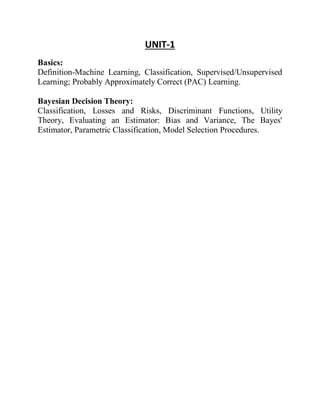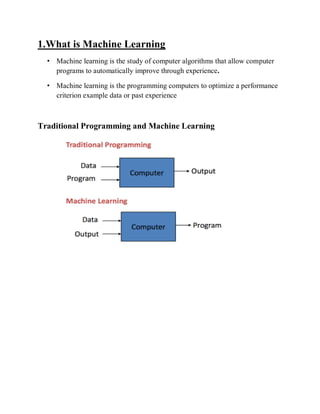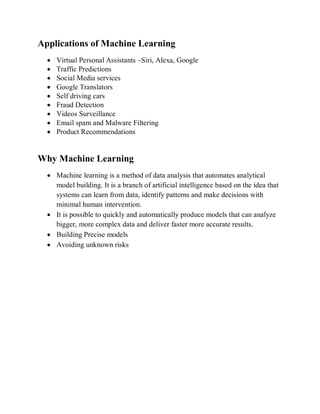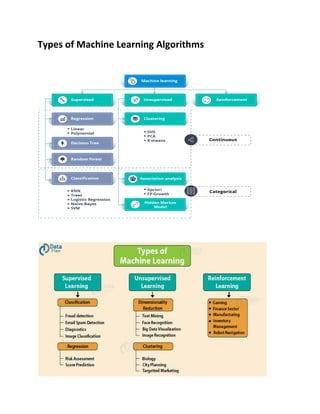Publicité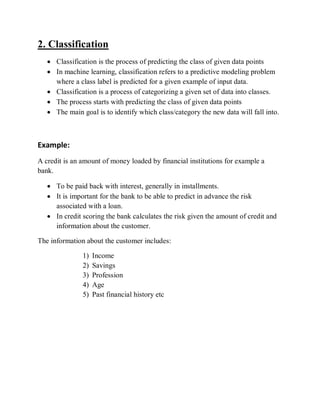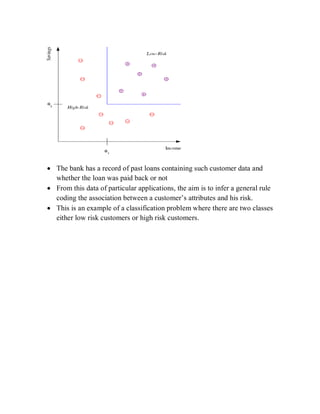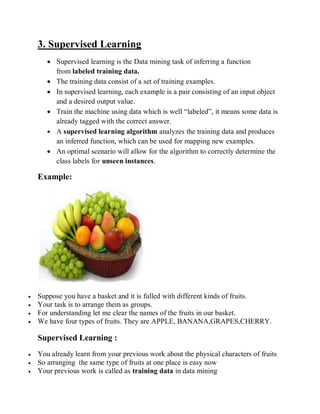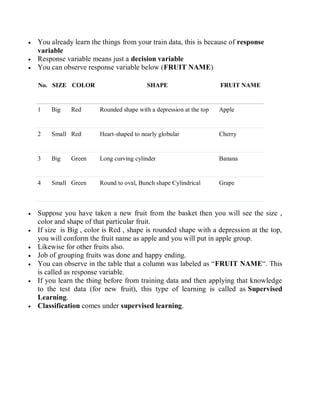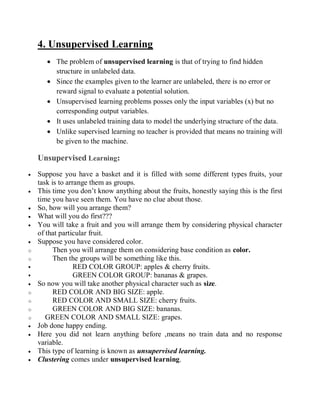Publicité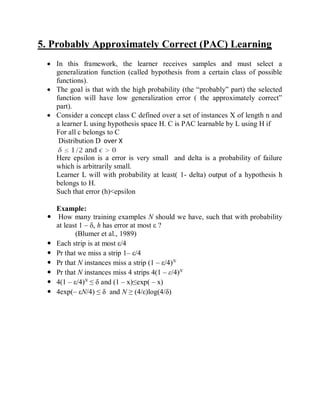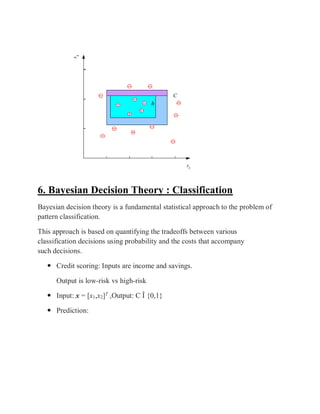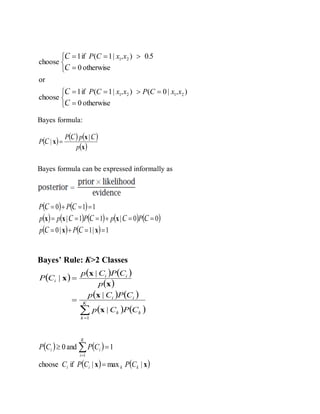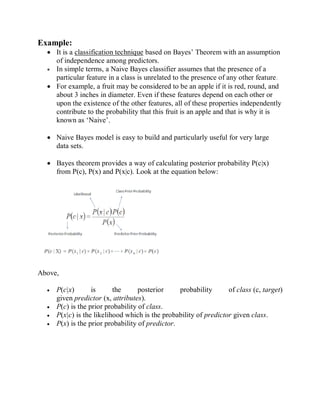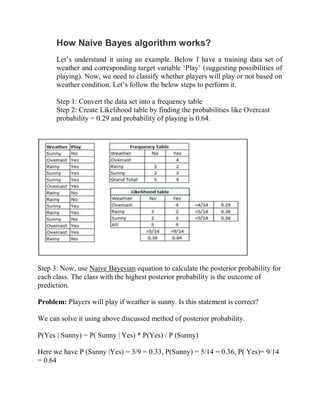Publicité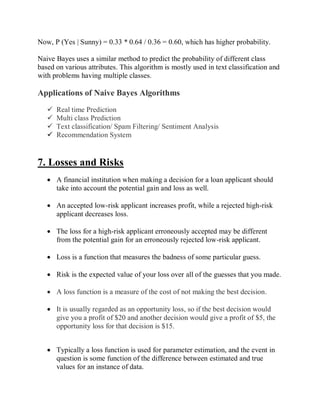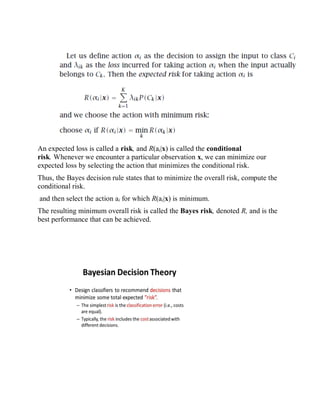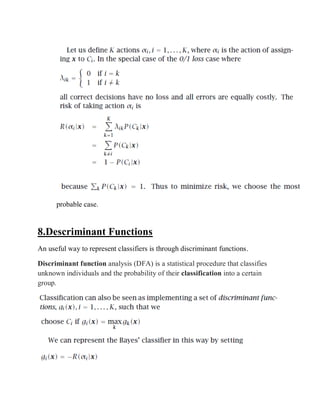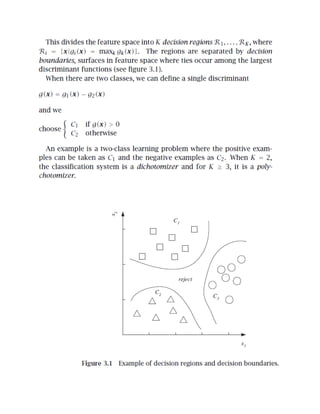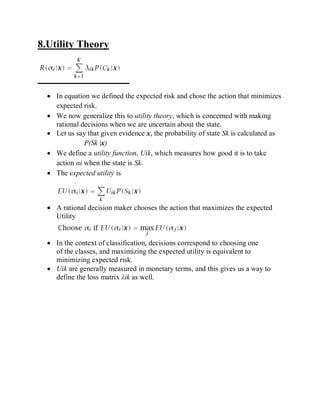Publicité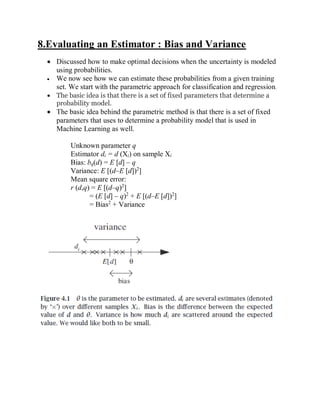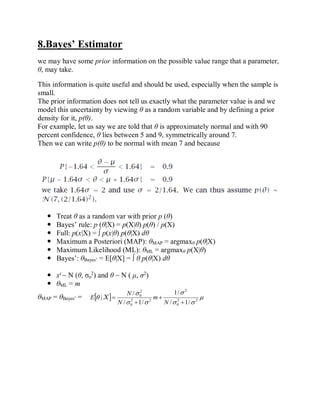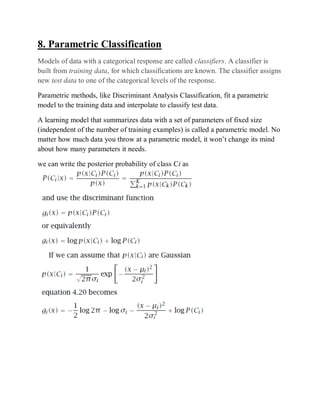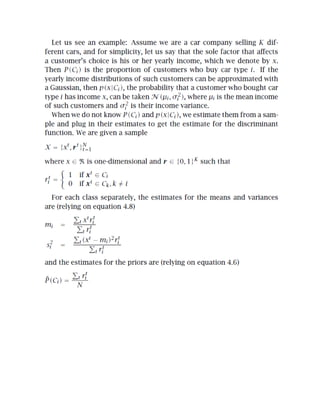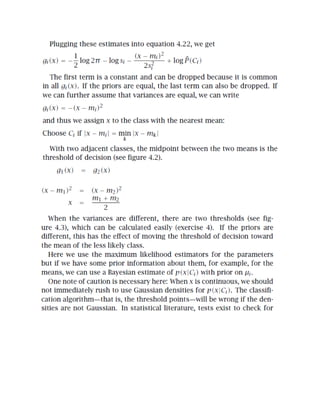Publicité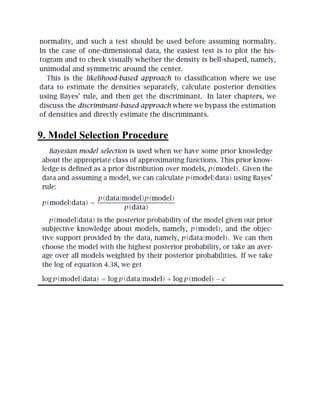Prochain SlideShare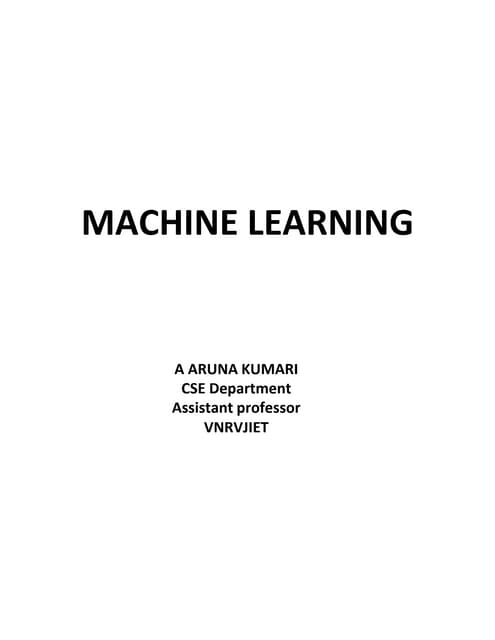Supervised learning and unsupervised learning
Chargement dans ... 3
1 sur 25
Publicité

### Unit-1.pdf

1. UNIT-1 Basics: Definition-Machine Learning, Classification, Supervised/Unsupervised Learning; Probably Approximately Correct (PAC) Learning. Bayesian Decision Theory: Classification, Losses and Risks, Discriminant Functions, Utility Theory, Evaluating an Estimator: Bias and Variance, The Bayes' Estimator, Parametric Classification, Model Selection Procedures.
2. 1.What is Machine Learning • Machine learning is the study of computer algorithms that allow computer programs to automatically improve through experience. • Machine learning is the programming computers to optimize a performance criterion example data or past experience Traditional Programming and Machine Learning
3. Applications of Machine Learning  Virtual Personal Assistants –Siri, Alexa, Google  Traffic Predictions  Social Media services  Google Translators  Self driving cars  Fraud Detection  Videos Surveillance  Email spam and Malware Filtering  Product Recommendations Why Machine Learning  Machine learning is a method of data analysis that automates analytical model building. It is a branch of artificial intelligence based on the idea that systems can learn from data, identify patterns and make decisions with minimal human intervention.  It is possible to quickly and automatically produce models that can analyze bigger, more complex data and deliver faster more accurate results.  Building Precise models  Avoiding unknown risks
4. Types of Machine Learning Algorithms
5. 2. Classification  Classification is the process of predicting the class of given data points  In machine learning, classification refers to a predictive modeling problem where a class label is predicted for a given example of input data.  Classification is a process of categorizing a given set of data into classes.  The process starts with predicting the class of given data points  The main goal is to identify which class/category the new data will fall into. Example: A credit is an amount of money loaded by financial institutions for example a bank.  To be paid back with interest, generally in installments.  It is important for the bank to be able to predict in advance the risk associated with a loan.  In credit scoring the bank calculates the risk given the amount of credit and information about the customer. The information about the customer includes: 1) Income 2) Savings 3) Profession 4) Age 5) Past financial history etc
6.  The bank has a record of past loans containing such customer data and whether the loan was paid back or not  From this data of particular applications, the aim is to infer a general rule coding the association between a customer’s attributes and his risk.  This is an example of a classification problem where there are two classes either low risk customers or high risk customers.
8.  You already learn the things from your train data, this is because of response variable  Response variable means just a decision variable  You can observe response variable below (FRUIT NAME) No. SIZE COLOR SHAPE FRUIT NAME 1 Big Red Rounded shape with a depression at the top Apple 2 Small Red Heart-shaped to nearly globular Cherry 3 Big Green Long curving cylinder Banana 4 Small Green Round to oval, Bunch shape Cylindrical Grape  Suppose you have taken a new fruit from the basket then you will see the size , color and shape of that particular fruit.  If size is Big , color is Red , shape is rounded shape with a depression at the top, you will conform the fruit name as apple and you will put in apple group.  Likewise for other fruits also.  Job of grouping fruits was done and happy ending.  You can observe in the table that a column was labeled as “FRUIT NAME“. This is called as response variable.  If you learn the thing before from training data and then applying that knowledge to the test data (for new fruit), this type of learning is called as Supervised Learning.  Classification comes under supervised learning.
9. 4. Unsupervised Learning  The problem of unsupervised learning is that of trying to find hidden structure in unlabeled data.  Since the examples given to the learner are unlabeled, there is no error or reward signal to evaluate a potential solution.  Unsupervised learning problems posses only the input variables (x) but no corresponding output variables.  It uses unlabeled training data to model the underlying structure of the data.  Unlike supervised learning no teacher is provided that means no training will be given to the machine. Unsupervised Learning:  Suppose you have a basket and it is filled with some different types fruits, your task is to arrange them as groups.  This time you don’t know anything about the fruits, honestly saying this is the first time you have seen them. You have no clue about those.  So, how will you arrange them?  What will you do first???  You will take a fruit and you will arrange them by considering physical character of that particular fruit.  Suppose you have considered color. o Then you will arrange them on considering base condition as color. o Then the groups will be something like this.  RED COLOR GROUP: apples & cherry fruits.  GREEN COLOR GROUP: bananas & grapes.  So now you will take another physical character such as size. o RED COLOR AND BIG SIZE: apple. o RED COLOR AND SMALL SIZE: cherry fruits. o GREEN COLOR AND BIG SIZE: bananas. o GREEN COLOR AND SMALL SIZE: grapes.  Job done happy ending.  Here you did not learn anything before ,means no train data and no response variable.  This type of learning is known as unsupervised learning.  Clustering comes under unsupervised learning.
10. 5. Probably Approximately Correct (PAC) Learning  In this framework, the learner receives samples and must select a generalization function (called hypothesis from a certain class of possible functions).  The goal is that with the high probability (the “probably” part) the selected function will have low generalization error ( the approximately correct” part).  Consider a concept class C defined over a set of instances X of length n and a learner L using hypothesis space H. C is PAC learnable by L using H if For all c belongs to C Distribution D over X Here epsilon is a error is very small and delta is a probability of failure which is arbitrarily small. Learner L will with probability at least( 1- delta) output of a hypothesis h belongs to H. Such that error (h)<epsilon Example:  How many training examples N should we have, such that with probability at least 1 ‒ δ, h has error at most ε ? (Blumer et al., 1989)  Each strip is at most ε/4  Pr that we miss a strip 1‒ ε/4  Pr that N instances miss a strip (1 ‒ ε/4)N  Pr that N instances miss 4 strips 4(1 ‒ ε/4)N  4(1 ‒ ε/4)N ≤ δ and (1 ‒ x)≤exp( ‒ x)  4exp(‒ εN/4) ≤ δ and N ≥ (4/ε)log(4/δ)
11. 6. Bayesian Decision Theory : Classification Bayesian decision theory is a fundamental statistical approach to the problem of pattern classification. This approach is based on quantifying the tradeoffs between various classification decisions using probability and the costs that accompany such decisions.  Credit scoring: Inputs are income and savings. Output is low-risk vs high-risk  Input: x = [x1,x2]T ,Output: C Î {0,1}  Prediction:
12.                otherwise 0 ) | 0 ( ) | 1 ( if 1 choose or otherwise 0 5 0 ) | 1 ( if 1 choose 2 1 2 1 2 1 C C C C ,x x C P ,x x C P . ,x x C P Bayes formula:         x x x p p P P C C C | |  Bayes formula can be expressed informally as                   1 | 1 | 0 0 0 | 1 1 | 1 1 0               x x x x x C C C C C C C C P p P p P p p P P Bayes’ Rule: K>2 Classes                     K k k k i i i i i C P C p C P C p p C P C p C P 1 | | | | x x x x x         x x | max | if choose 1 and 0 1 k k i i K i i i C P C P C C P C P     
13. Example:  It is a classification technique based on Bayes’ Theorem with an assumption of independence among predictors.  In simple terms, a Naive Bayes classifier assumes that the presence of a particular feature in a class is unrelated to the presence of any other feature.  For example, a fruit may be considered to be an apple if it is red, round, and about 3 inches in diameter. Even if these features depend on each other or upon the existence of the other features, all of these properties independently contribute to the probability that this fruit is an apple and that is why it is known as ‘Naive’.  Naive Bayes model is easy to build and particularly useful for very large data sets.  Bayes theorem provides a way of calculating posterior probability P(c|x) from P(c), P(x) and P(x|c). Look at the equation below: Above,  P(c|x) is the posterior probability of class (c, target) given predictor (x, attributes).  P(c) is the prior probability of class.  P(x|c) is the likelihood which is the probability of predictor given class.  P(x) is the prior probability of predictor.
14. How Naive Bayes algorithm works? Let’s understand it using an example. Below I have a training data set of weather and corresponding target variable ‘Play’ (suggesting possibilities of playing). Now, we need to classify whether players will play or not based on weather condition. Let’s follow the below steps to perform it. Step 1: Convert the data set into a frequency table Step 2: Create Likelihood table by finding the probabilities like Overcast probability = 0.29 and probability of playing is 0.64. Step 3: Now, use Naive Bayesian equation to calculate the posterior probability for each class. The class with the highest posterior probability is the outcome of prediction. Problem: Players will play if weather is sunny. Is this statement is correct? We can solve it using above discussed method of posterior probability. P(Yes | Sunny) = P( Sunny | Yes) * P(Yes) / P (Sunny) Here we have P (Sunny |Yes) = 3/9 = 0.33, P(Sunny) = 5/14 = 0.36, P( Yes)= 9/14 = 0.64
15. Now, P (Yes | Sunny) = 0.33 * 0.64 / 0.36 = 0.60, which has higher probability. Naive Bayes uses a similar method to predict the probability of different class based on various attributes. This algorithm is mostly used in text classification and with problems having multiple classes. Applications of Naive Bayes Algorithms  Real time Prediction  Multi class Prediction  Text classification/ Spam Filtering/ Sentiment Analysis  Recommendation System 7. Losses and Risks  A financial institution when making a decision for a loan applicant should take into account the potential gain and loss as well.  An accepted low-risk applicant increases profit, while a rejected high-risk applicant decreases loss.  The loss for a high-risk applicant erroneously accepted may be different from the potential gain for an erroneously rejected low-risk applicant.  Loss is a function that measures the badness of some particular guess.  Risk is the expected value of your loss over all of the guesses that you made.  A loss function is a measure of the cost of not making the best decision.  It is usually regarded as an opportunity loss, so if the best decision would give you a profit of \$20 and another decision would give a profit of \$5, the opportunity loss for that decision is \$15.  Typically a loss function is used for parameter estimation, and the event in question is some function of the difference between estimated and true values for an instance of data.
16. An expected loss is called a risk, and R(ai|x) is called the conditional risk. Whenever we encounter a particular observation x, we can minimize our expected loss by selecting the action that minimizes the conditional risk. Thus, the Bayes decision rule states that to minimize the overall risk, compute the conditional risk. and then select the action ai for which R(ai|x) is minimum. The resulting minimum overall risk is called the Bayes risk, denoted R, and is the best performance that can be achieved.
17. probable case. 8.Descriminant Functions An useful way to represent classifiers is through discriminant functions. Discriminant function analysis (DFA) is a statistical procedure that classifies unknown individuals and the probability of their classification into a certain group.
18. 8.Utility Theory  In equation we defined the expected risk and chose the action that minimizes expected risk.  We now generalize this to utility theory, which is concerned with making rational decisions when we are uncertain about the state.  Let us say that given evidence x, the probability of state Sk is calculated as P(Sk |x)  We define a utility function, Uik, which measures how good it is to take action αi when the state is Sk.  The expected utility is  A rational decision maker chooses the action that maximizes the expected Utility  In the context of classification, decisions correspond to choosing one of the classes, and maximizing the expected utility is equivalent to minimizing expected risk.  Uik are generally measured in monetary terms, and this gives us a way to define the loss matrix λik as well.
19. 8.Evaluating an Estimator : Bias and Variance  Discussed how to make optimal decisions when the uncertainty is modeled using probabilities.  We now see how we can estimate these probabilities from a given training set. We start with the parametric approach for classification and regression.  The basic idea is that there is a set of fixed parameters that determine a probability model.  The basic idea behind the parametric method is that there is a set of fixed parameters that uses to determine a probability model that is used in Machine Learning as well. Unknown parameter q Estimator di = d (Xi) on sample Xi Bias: bq(d) = E [d] – q Variance: E [(d–E [d])2 ] Mean square error: r (d,q) = E [(d–q)2 ] = (E [d] – q)2 + E [(d–E [d])2 ] = Bias2 + Variance
20. 8.Bayes’ Estimator we may have some prior information on the possible value range that a parameter, θ, may take. This information is quite useful and should be used, especially when the sample is small. The prior information does not tell us exactly what the parameter value is and we model this uncertainty by viewing θ as a random variable and by defining a prior density for it, p(θ). For example, let us say we are told that θ is approximately normal and with 90 percent confidence, θ lies between 5 and 9, symmetrically around 7. Then we can write p(θ) to be normal with mean 7 and because  Treat θ as a random var with prior p (θ)  Bayes’ rule: p (θ|X) = p(X|θ) p(θ) / p(X)  Full: p(x|X) = ∫ p(x|θ) p(θ|X) dθ  Maximum a Posteriori (MAP): θMAP = argmaxθ p(θ|X)  Maximum Likelihood (ML): θML = argmaxθ p(X|θ)  Bayes’: θBayes’ = E[θ|X] = ∫ θ p(θ|X) dθ  xt ~ N (θ, σo 2 ) and θ ~ N ( μ, σ2 )  θML = m θMAP = θBayes’ =           2 2 0 2 2 2 0 2 0 / 1 / / 1 / 1 / / |     N m N N E X
21. 8. Parametric Classification Models of data with a categorical response are called classifiers. A classifier is built from training data, for which classifications are known. The classifier assigns new test data to one of the categorical levels of the response. Parametric methods, like Discriminant Analysis Classification, fit a parametric model to the training data and interpolate to classify test data. A learning model that summarizes data with a set of parameters of fixed size (independent of the number of training examples) is called a parametric model. No matter how much data you throw at a parametric model, it won’t change its mind about how many parameters it needs. we can write the posterior probability of class Ci as
22. 9. Model Selection Procedure
Publicité